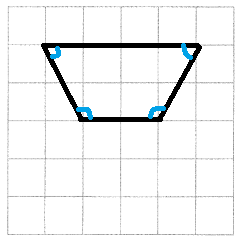# Math in Focus Grade 3 Chapter 17 Practice 1 Answer Key Understanding and Identifying Angles

This handy Math in Focus Grade 3 Workbook Answer Key Chapter 17 Practice 1 Understanding and Identifying Angles provides detailed solutions for the textbook questions.

## Math in Focus Grade 3 Chapter 17 Practice 1 Answer Key Understanding and Identifying Angles

Name each figure as a point, line, or line segment.

Example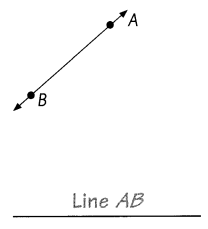Question 1.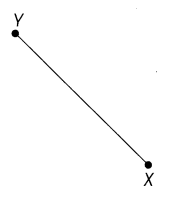Line segment XY
Explanation:
A line segment is a part of a line that is bounded by two distinct end points,
and contains every point on the line that is between its endpoints.

Question 2.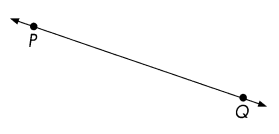Line PQ
Explanation:
A line has no end points.
A line segment has two endpoints.
A line segment connects both endpoints.
If the two lines cannot meet at any point, they are called parallel lines.

Question 3.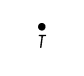Point T
Explanation:
A point is a location represented by a dot.
A point does not have any length, width, shape or size, it only has a position.
When two distinct points are connected they form a line.

Check (✓) the statements that are true.

Question 4.
A line segment is part of a line.
Explanation:
A line segment is a part of a line that is bounded by two distinct end points,
and contains every point on the line that is between its endpoints.

Question 5.
A point is an exact location in space.
Explanation:
A point is a location represented by a dot.
A point does not have any length, width, shape or size, it only has a position.
When two distinct points are connected they form a line.

Question 6.
A line has two endpoints.
Explanation:
A line has no end points.
A line segment has two endpoints.
A line segment connects both endpoints.
If the two lines cannot meet at any point, they are called parallel lines..

Check (✓) the box if an angle is shown.

Question 7.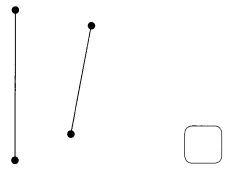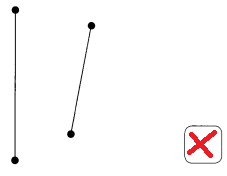Explanation:
The above figure doesn’t contain any angle,
they are line segments.

Question 8.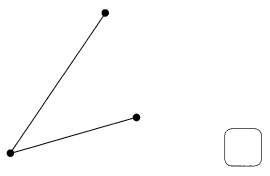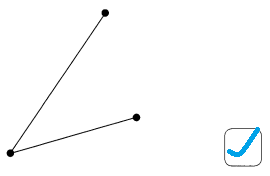Explanation:
The above figure contain angle greater than right angle.
So, it is Obtuse angle.

Question 9.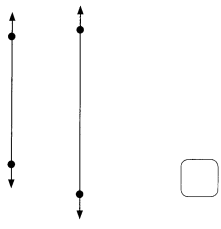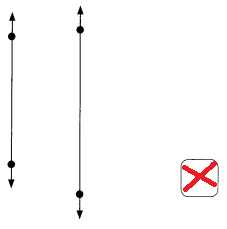Explanation:
The above figure doesn’t contain any angle,
they are line segments.

Question 10.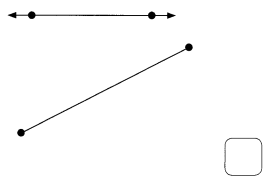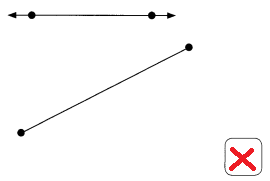Explanation:
The above figure doesn’t contain any angle,
they are perpendicular line segments.

Question 11.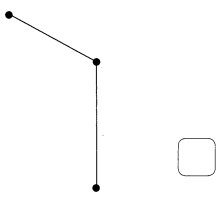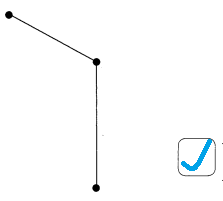Explanation:
The above figure contain angle less than right angle.
So, it is Acute angle.

Question 12.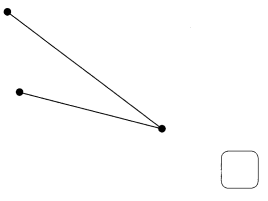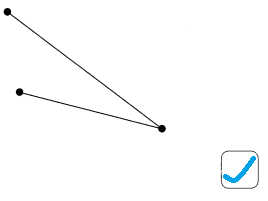Explanation:
The above figure contain angle less than right angle.
So, it is Acute angle.

Mark two angles on each object.

Question 13.Explanation:
The above figure is in rectangular shape,
it has two angles, with 90 degrees.
So, the angles are right angle triangles.

Question 14.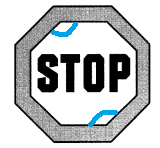Explanation:
The above figure is in Octagon,
Octagon is a polygon in geometry, which has 8 sides and 8 angles.
That means the number of vertices is 8 and the number of edges is 8.
So, octagon is a 8-sided polygon and it is a two dimensional plane figure.
All the sides are joined with each other end-to-end to form a shape.

Question 15.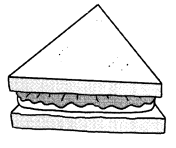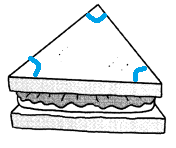Explanation:
The above figure is in Triangle.
A triangle has three corners,
3 angles sum to 180 degrees.

Question 16.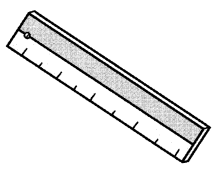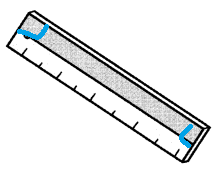Explanation:
The above figure is in rectangular shape,
it has two angles, with 90 degrees.
So, the angles are right angle triangles.

Question 17.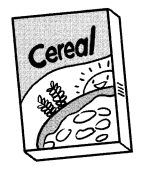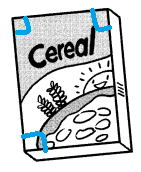Explanation:
The above figure book is in rectangular shape,
it has two angles, with 90 degrees.
So, the angles are right angle triangles.

Question 18.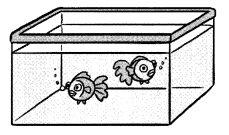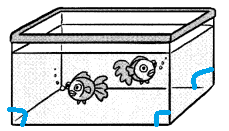Explanation:
The above figure aquarium has 3 angles.

Mark two angles in each plane shape if possible.

Question 19.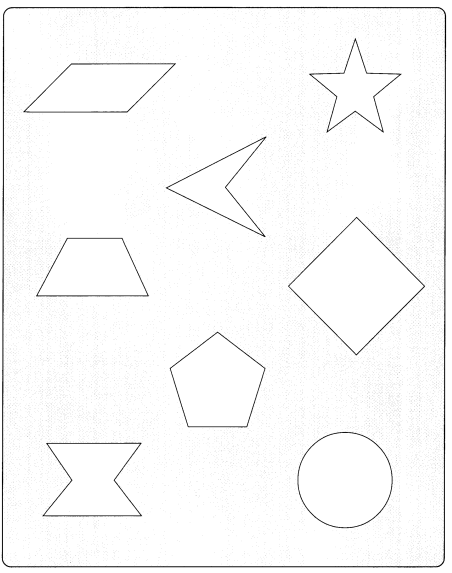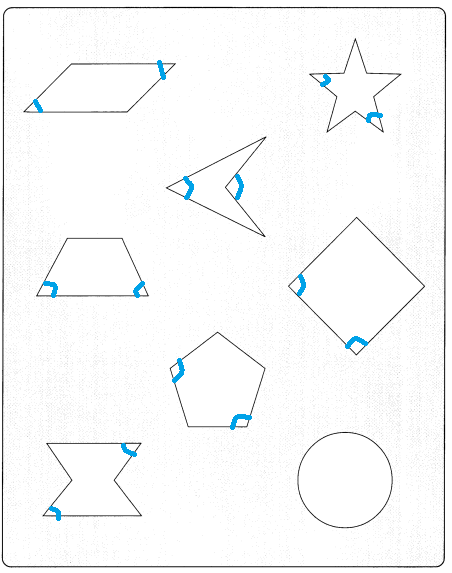Explanation:
In the above figure expect circle all the figures have 2 angles,
as the circle does not contain any angles.

Complete the table. Mark all the angles in each plane shape.

Question 20.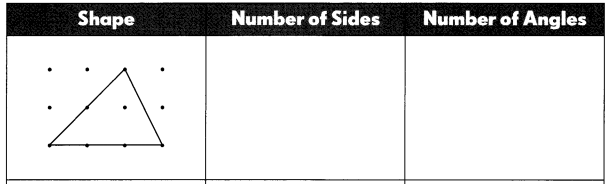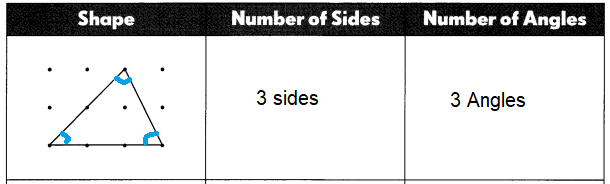Explanation:
The above figure is Triangle.
A triangle has three sides,
3 angles sum to 180 degrees.

Question 21.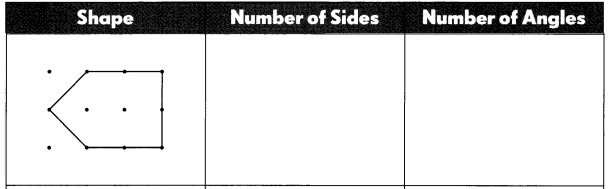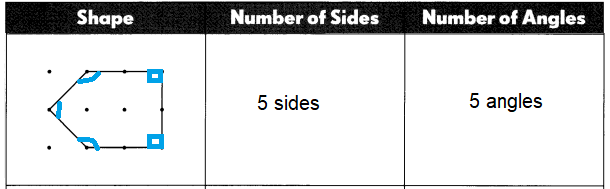Explanation:
A pentagon is a five-sided polygon with five straight sides and five interior angles that sum up to 540°540°.
A pentagon shape is a plane figure, or flat (two-dimensional) 5-sided geometric shape.

Question 22.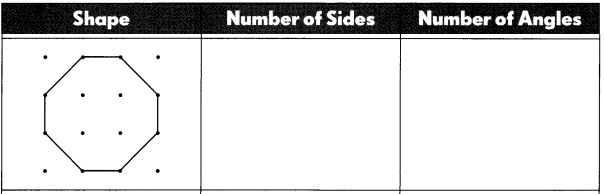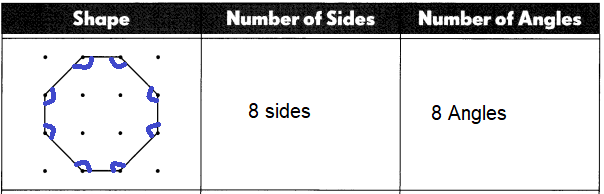Explanation:
The above figure is in Octagon,
Octagon is a polygon in geometry, which has 8 sides and 8 angles.
That means the number of vertices is 8 and the number of edges is 8.
So, octagon is a 8-sided polygon and it is a two dimensional plane figure.
All the sides are joined with each other end-to-end to form a shape.

Question 23.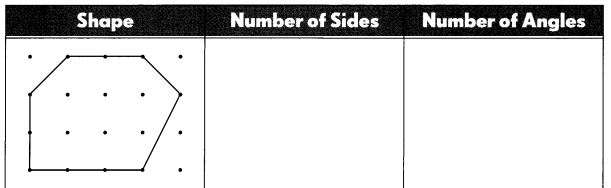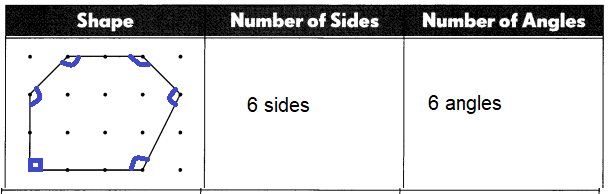Explanation:
In geometry, a hexagon can be defined as a polygon with six sides.
The two-dimensional shape has 6 sides, 6 vertices and 6 angles.

Question 24.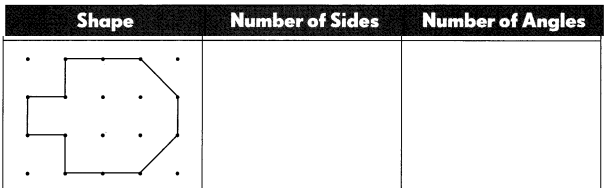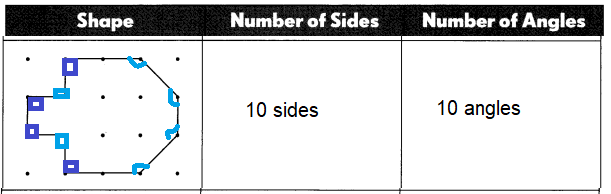Explanation:
A polygon with 10 sides and 10 angles and 10 vertices is called as Decagon.

Draw four different plane shapes. Write the number of sides and angles in each shape.

Question 25.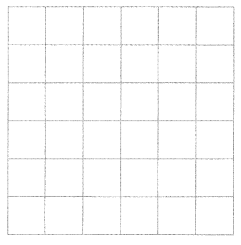___________ sides _________ angles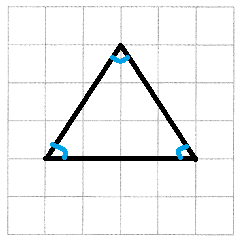Triangle
3 sides
3 angles
Explanation:
The above figure is Triangle.
A triangle has three sides,
3 angles sum to 180 degrees.

Question 26.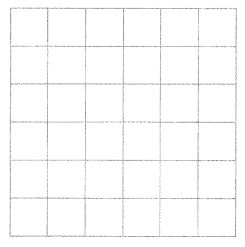___________ sides _________ angles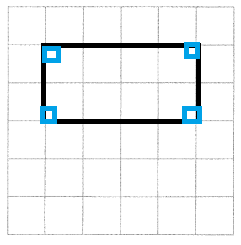Rectangle
4 sides
4 angles
Explanation:
The above figure is in rectangular shape,
it has four sides and four angles, with 90 degrees.
And the angles are right angle triangles.

Question 27.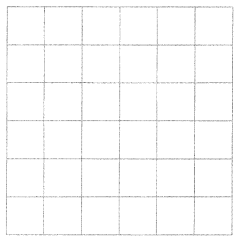___________ sides _________ angles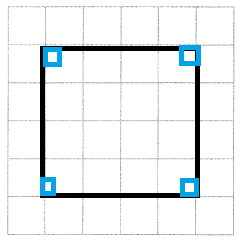Square
4 sides
4 angles
Explanation:
A Square is a regular quadrilateral,
which has all the four sides of equal length and,
all four angles. with right angle triangles.

Question 28.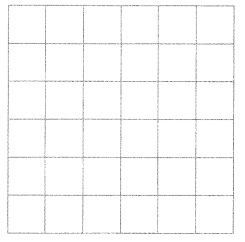___________ sides _________ angles